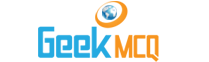# Engineering :: Strength of Materials

1.  Whenever some external system of forces acts on a body, it undergoes some deformation. As the body undergoes some deformation, it sets up some resistance to the deformation. This resistance per unit area to deformation, is called
 A. strain B. stress C. pressure D. modulus of elasticity

2.  The unit of stress in S.I. units is
 A. N / mm2 B. kN / mm2 C. N / m2 D. any one of these

3.  The deformation per unit length is called
 A. tensile stress B. compressive stress C. shear stress D. strain

4.  When a body is subjected to two equal and opposite pushes, as a result of which the body tends to reduce its length, the stress and strain induced is compressive.
 A. True B. False

5.  When a body is subjected to two equal and opposite pulls, as a result of which the body tends to extend its length, the stress and strain induced is
 A. compressive stress, tensile strain B. tensile stress, compressive strain C. tensile stress, tensile strain D. compressive stress, compressive strain

6.  When a body is subjected to two equal and opposite forces, acting tangentially across the resisting section, as a result of which the body tends to shear off across the section, the stress and strain induced is
 A. tensile stress, tensile strain B. compressive stress, compressive strain C. shear stress, tensile strain D. shear stress, shear strain

7.  Which of the following is a proper sequence?
 A. proportional limit, elastic limit, yielding, failure B. elastic limit, proportional limit, yielding, failure C. yielding, proportional limit, elastic limit, failure D. none of the above

8.  Hook's law holds good up to
 A. yield point B. elastic limit C. plastic limit D. breaking point

9.  Whenever a material is loaded within elastic limit, stress is
 A. equal to B. directly proportional to C. inversely proportional to

10.  The ratio of linear stress to the linear strain is called
 A. modulus of rigidity B. modulus of elasticity C. bulk modulus D. Poisson's ratio